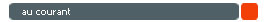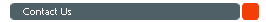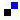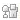Home About Us Practice Test GMAT eBooks Math Books GMAT Classes Forums B SchoolsGMAT Frequently Asked Questions GMAT CAT Test Details / Signup GMAT Syllabus, Testing Subject areas GMAT Math Syllabus, Sample QuestionseBook on Number Theory and Inequalities eBook on Permutation & Combination eBook on Geometry Doubts, discussions in GMAT Math Share your GMAT Test experience 4GMAT's Achievers' List TestimonialsFree GMAT Questions Answers Free GMAT Math Test GMAT Blogs GMAT Quant Blogs GMAT FAQsTop US B Schools R1/R2 deadlines Featured B School Rearranging numbers - Permutation Counting number of 1s and 0sChennai : 95000 48484 Mumbai : 80970 48484 Bangalore : 91419 48484 eMail : info@4gmat.com# Quantitative Ability - Math Problem Solving

The Quantitative section of the GMAT Test measures basic mathematical skills. It tests a candidate's understanding of elementary concepts in mathematics learnt at school and the ability to reason quantitatively. The section involves solving quantitative problems, reasoning using quantitative techniques and interpreting graphic data.

Preparing for the GMAT Quant section would involve learning and mastering concepts in
• Arithmetic
• Elementary Algebra and
• Basic Geometry
Here is a list of topics in Math that a candidate should include in his or her GMAT preparation plan. The list is only a representative indicator of what needs to be prepared for and does not include all areas tested in GMAT.

Topics in Arithmetic

Number Systems & Number Theory; Percentages; Profit & Loss; Simple & Compound Interest; Speed, Time & Distance; Pipes, Cisterns & Work Time; Simple & Weighted Averages; Ratio, Proportion & Variation; Mixtures & Alligation.

Topics in Algebra / Modern Math

Permutation & Combination; Probability; Arithmetic & Geometric Progression; Quadratic Equations; Set Theory; Inequalities and Basic statistics.

Topics in Geometry

Basic concepts in Geometry; Coordinate Geometry and Mensuration.

Sample GMAT Problem Solving Question

A sample GMAT Problem solving question along with the instructions that typically precede a problem solving question is available by clicking on the read more icon.GMAT Data Sufficiency
About third of the questions in the GMAT Quantitative Section comprising 37 questions are data sufficiency questions.Add to del.icio.usStumble Itdigg this